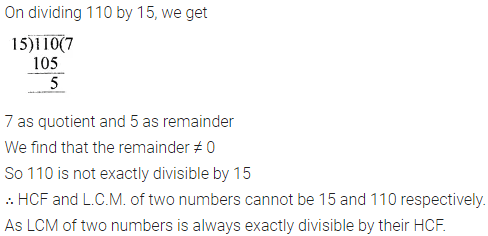# ML Aggarwal Class 6 Solutions for ICSE Maths Chapter 4 Playing with Numbers Ex 4.5

## ML Aggarwal Class 6 Solutions for ICSE Maths Chapter 4 Playing with Numbers Ex 4.5

Question 1.
Find the L.C.M. of the given numbers by prime factorisation method :
(i) 28, 98
(ii) 36, 40, 126
(iii) 108, 135, 162
(iv) 24, 28, 196.
Solution: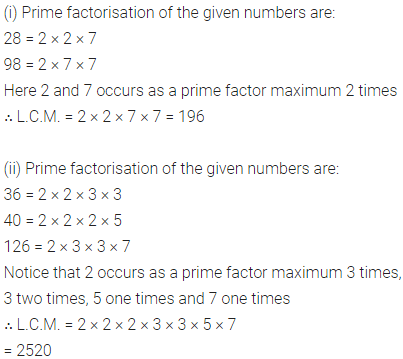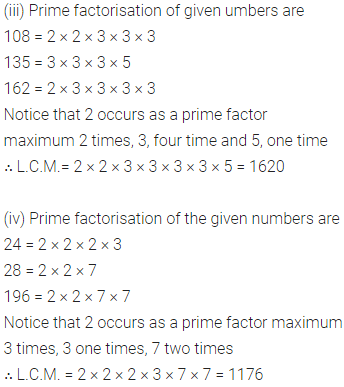Question 2.
Find the L.C.M. of the given numbers by division method :
(i) 480, 672
(ii) 6, 8, 45
(iii) 24, 40, 84
(iv) 20, 36, 63, 67
Solution: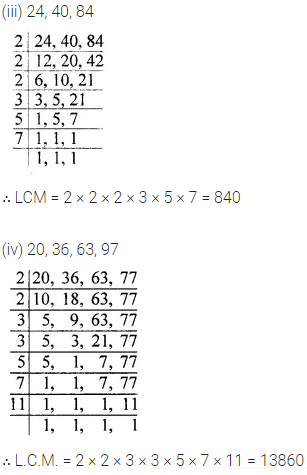Question 3.
Find the least number which when increased by 15 is exactly divisible by 15, 35 and 48.
Solution: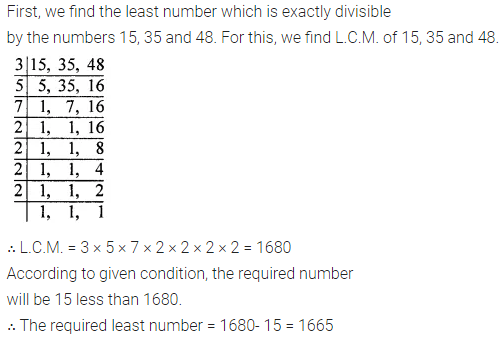Question 4.
Find the least number which when divided by 6, 15 and 18 leaves remainder 5 in each case.
Solution:Question 5.
Find the least number which when divided by 24, 36, 45 and 54 leaves a remainder of 3 in each case.
Solution: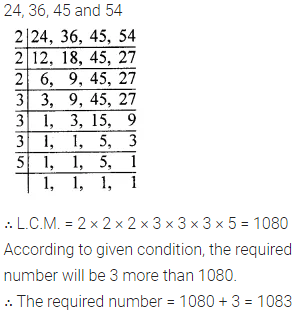Question 6.
Find the greatest 3-digit number which is exactly divisible by 8, 20 and 24.
Solution:Question 7.
Find the smallest 4-digit number which is exactly divisible by 32, 36 and 48.
Solution: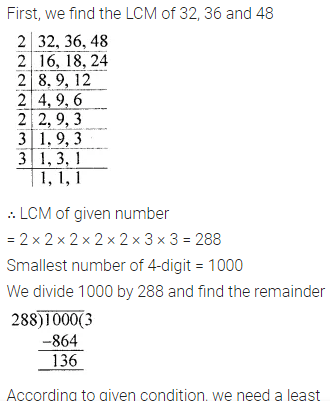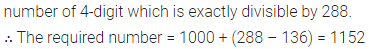Question 8.
Find the greatest 4-digit number which is exactly divisible by each of 8, 12 and 20.
Solution: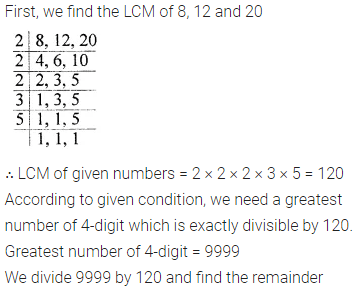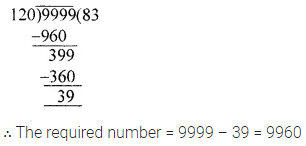Question 9.
Find the least number of five digits which is exactly divisible by 32, 36 and 45.
Solution: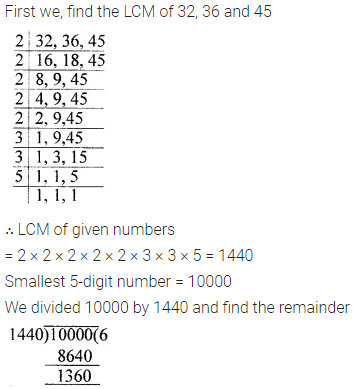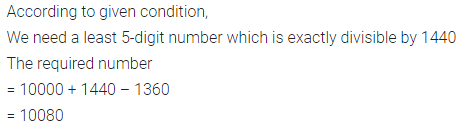Question 10.
Three boys step off together from the same spot. Their steps measure 63 cm, 70 cm and 77 cm respectively. What is the minimum distance each should cover so that all can cover the same distance in complete steps?
Solution: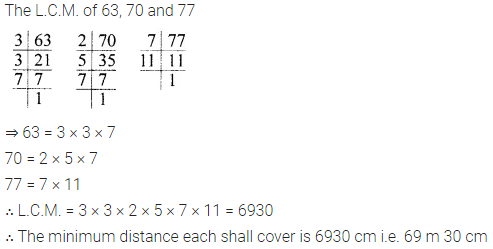Question 11.
Traffic lights at three different road crossing change after 48 seconds, 72 seconds and 108 seconds respectively. At what time will they change together again if they change simultaneously at 7 A.M.?
Solution: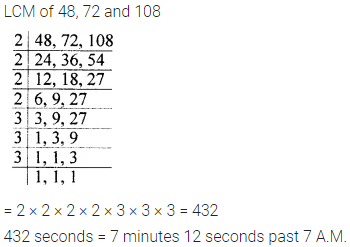Question 12.
If the product of two numbers is 4032 and their HCF is 12, find their LCM.
Solution: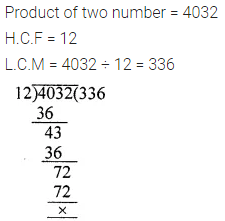Question 13.
The HCF and LCM of two numbers are 9 and 270 respectively. If one of the numbers is 45, find the other number.
Solution: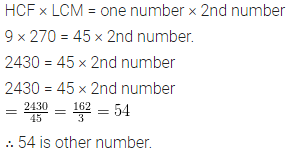Question 14.
Find the HCF of 180 and 336. Hence, find their LCM.
Solution: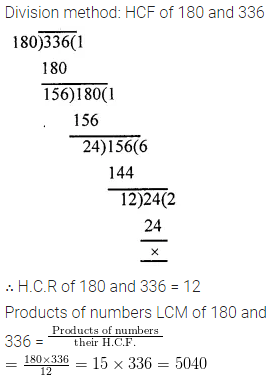Question 15.
Can two numbers have 15 as their HCF and 110 as their LCM? Give a reason to justify your answer.
Solution: